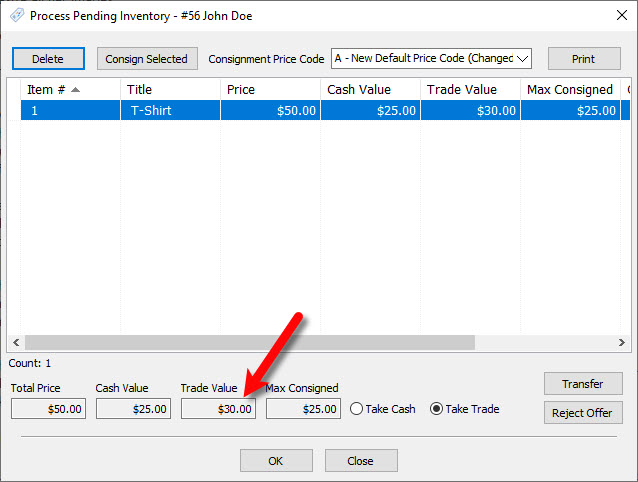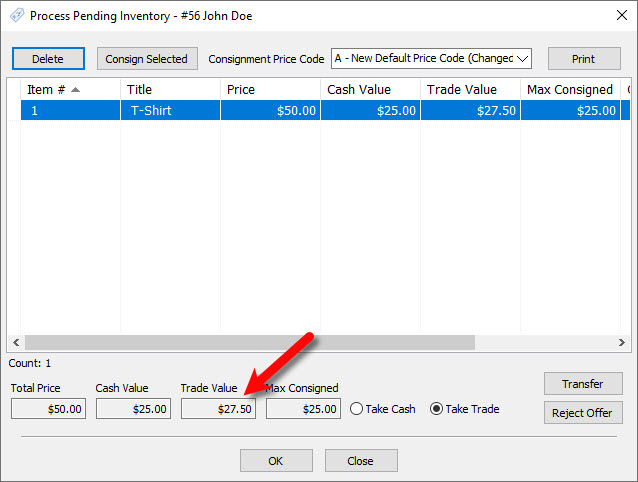# New Definitions for Multiplicative and Additive Trade Differential

Additive vs Multiplicative Differential Percentage For Trade

Differential Percentage For Trade Items: This will provide a bonus in store credit to the consignor when selecting to receive payment in the form of store credit over cash.

Additive: With this option enabled, if you set the differential to 10%, then the trade value would be the price of the item multiplied by the trade differential percentage added to the cash value.

Additive Example: You have an item that is priced at \$50.00.  Your split is 50/50, meaning the cash value of this item when processed would be \$25.00 to the client.  The  trade differential set to 10%, that would make the store credit trade offer \$30.00.

Price * Split percentage = Cash Value

Cash Value + Additional Trade Value = Total Trade Value

\$50 * 50% = \$25.00

\$50 * 10% = \$5.00

\$25 + \$5 = \$30.00

1.Price + Split Percentage = Cash Value

50 * .50 = \$25.00

2.Price * Differential Percentage = Additional Trade Value

50 * .10 = \$5.00

25 + 5 = \$30.00Multiplicative: With this option enabled, if you set the differential to 10%, then the trade value would be 110% the cash value.

Multiplicative Example: You have an item that is priced at \$50.00.  Your split is 50/50, meaning the cash value of this item when processed would be \$25.00 to the client.  With the trade differential set to 10%, that would make the store credit trade offer \$27.50.

Formula For Multiplicative Example:

Price * Split Percentage = Cash Value

Cash Value * Differential Percentage = Additional Trade Value

Additional Trade Value + Net to consignor = Total Trade value

\$50 * 50% = \$25

\$25 * 10% = \$2.50

\$2.50+ \$25.00 = \$27.50

1.Price * Split Percentage = Cash Value

50 * .50 = \$25.00

2.Cash Value * Differential Percentage = Additional Trade Value

25 * .10 = \$2.50

3.Additional Trade Value + Cash Value = Total Trade Value

2.50 + 25 = \$27.50